## Thermodynamics

Versión 1.1.1.0 (25.3 MB) por
This curriculum module contains interactive examples that teach fundamental concepts and basic terminology related to thermodynamics.

131 descargas

De GitHub

# Thermodynamics

Curriculum Module
Created with R2022b. Compatible with R2022b and later releases.

## Description

This curriculum module contains interactive MATLAB® live scripts that teach fundamental concepts and basic terminology related to introductory thermodynamics. Topics addressed include units, the first and second laws of thermodynamics, state variables, work, and the components of a refrigeration cycle.

You can use these live scripts as demonstrations in lectures, class activities, or interactive assignments outside class. This module consists of several interactive live scripts intended to introduce or deepen student understanding of thermodynamic concepts, including working with data tables and reading thermodynamic plots. Two additional scripts are included for instructors or students who want to go beyond the included material by installing CoolProp or building their own refrigerator model.

## Get started with the Thermodynamics interactive examples

Solutions are available upon instructor request. If you would like to request solutions or have a question, contact the MathWorks online teaching team.

## Prerequisite Domain Knowledge

These scripts assume background knowledge of common thermodynamic terminology as covered in secondary education such as temperature, pressure, kinetic energy, and potential energy as well as fluency with basic integral calculus. There is minimal MATLAB knowledge required for FirstLaw, SecondLaw and StatePlots, but you could use MATLAB Onramp as a resource to acquire familiarity with live scripts and MATLAB syntax. More familiarity with MATLAB is required for CreatePVplots, CalculateWork and Model_Fridge, as those are designed in Code Visible format. Familiarity with Simulink at the level of Simulink Onramp may be helpful for Model_Fridge as well.

For those interested in additional coding, SetUpCoolProp includes instructions for installing and accessing the CoolProp thermodynamic reference library. BuildRefrigerator walks through a project to build a refrigerator model in Simulink out of standard component blocks. BuildRefrigerator requires Simulink Onramp, at a minimum. If possible, Simscape Onramp would be helpful as well. Students are expected to find and insert specified blocks, connect blocks, edit block parameters, rename blocks, copy and paste subsystems, run simulations, and explore the output.

## Details

This script introduces the first law of thermodynamics with a focus on units, energy, systems, and conservation laws.

In this script, students will...

• Express various forms of energy in terms of fundamental units
• Apply the conservation of mass in simple, conceptual situations
• Identify systems with open and closed control volumes
• Apply the first law of thermodynamics

This script investigates pressure-volume (PV) diagrams and their relationship to state equations in a closed system.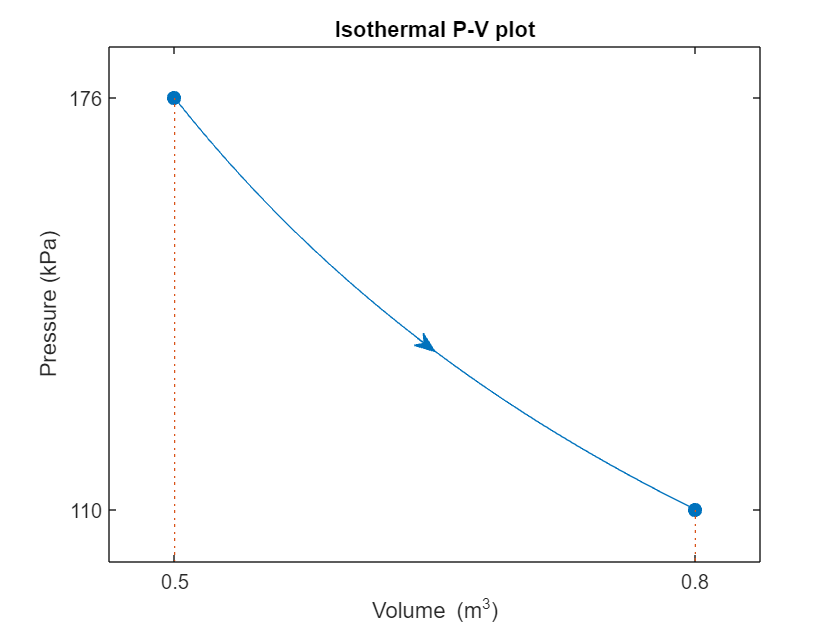In this script, students will...

• Identify key words including isothermal, isobaric, and isochoric, and translate word problems into thermodynamic terminology
• Identify and state necessary assumptions to express a thermodynamic relationship

This script introduces computing the work done by a system using analysis of its PV plot.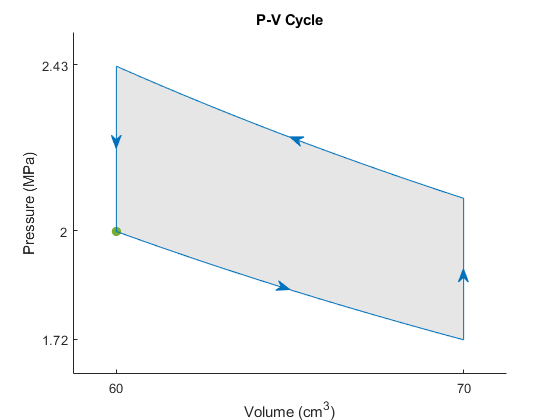In this script, students will...

• Identify positive, negative, and zero work from a P-V plot without computing the value
• Compute work in constant pressure, constant volume, and integrable P-V plots
• Compare various state equations to each other and to tabular data
• Compute the work done by a piston modeled in Simulink

This script introduces the second law of thermodynamics in both the Kelvin-Planck and Clausius formulations.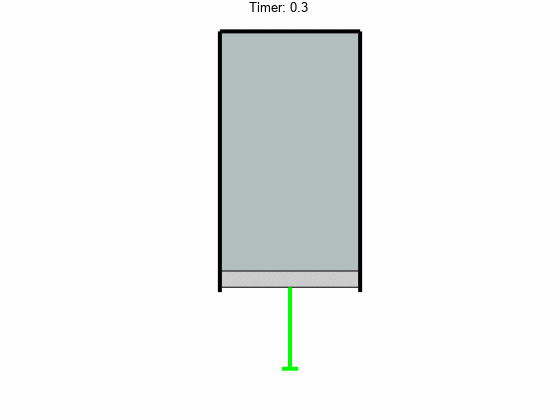In this script, students will...

• Identify thermal sources, sinks, and reservoirs
• Compute thermal efficiency
• Reflect on the second law of thermodynamics
• Use the universe as a system of reference for determining thermodynamic flows

This script introduces state diagrams and temperature-entropy plots.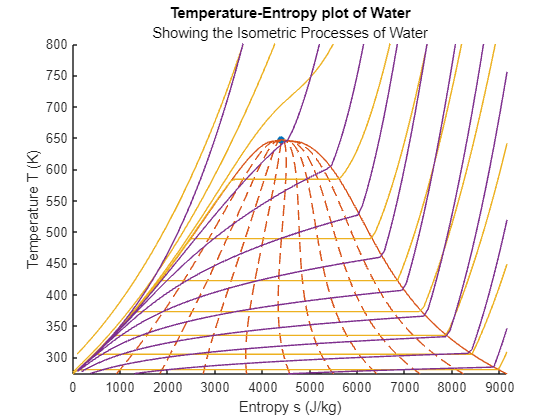In this script, students will...

• Investigate the standard shapes of state diagrams
• Investigate Temperature/Entropy plots for several pure substances including water, R134a, and ammonia
• Identify liquid, gas, two-phase, and supercritical states of matter on a temperature-entropy plot

Apply their knowledge of thermodynamics to a Simulink model of a real refrigerator.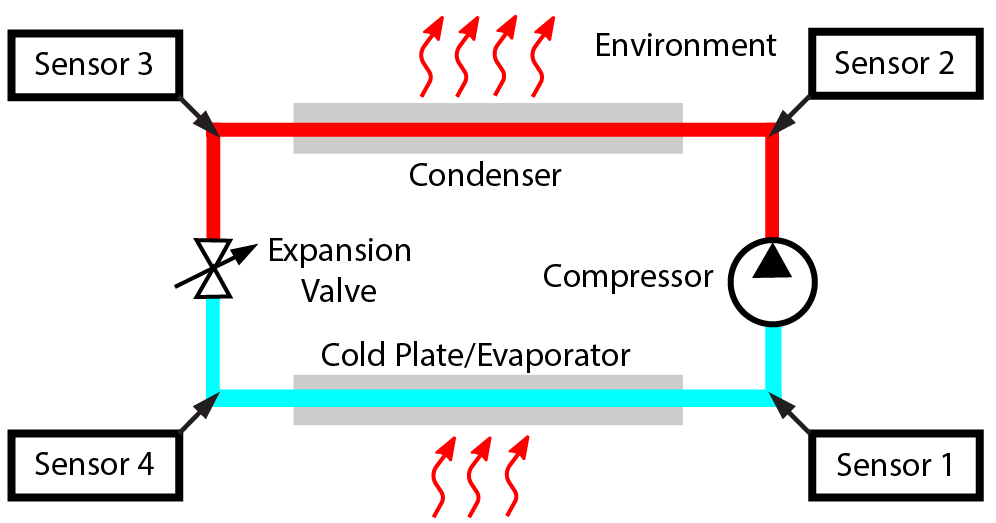In this script, students will...

• Apply understanding about thermodynamic cycles to model a refrigerator
• Perform unit conversions required to extract values from a database and use them in a model
• Read and interpret thermodynamic plots
• Create informative plots based on thermodynamic sensor data

## With More Coding

SetUpCoolProp.mlx This script is only appropriate for desktop versions of MATLAB.

In this script, students will...

Using Simulink and the capabilities of the Simscape Two-Phase Fluid libraries, build and test a complete refrigerator from a blank model.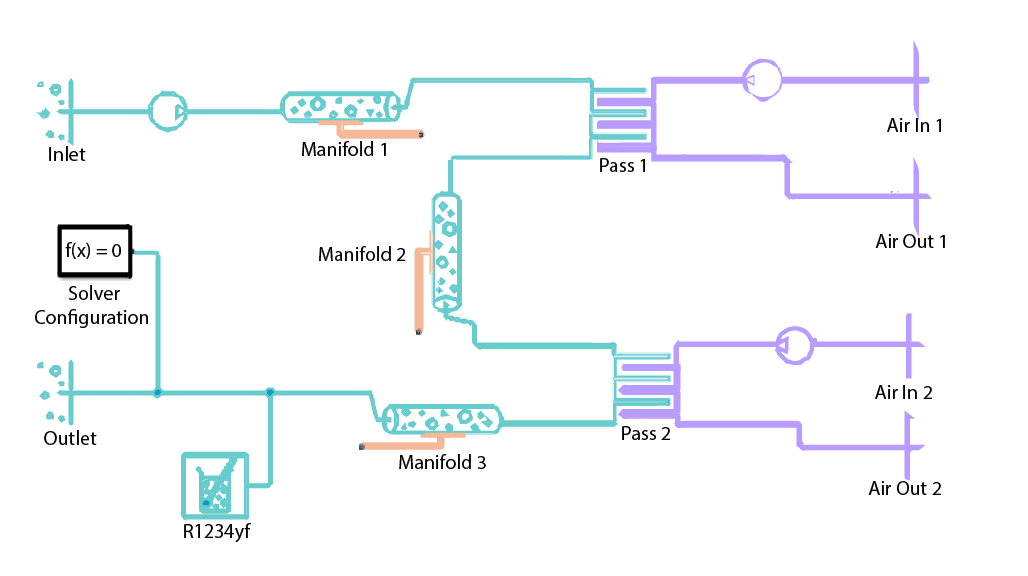In this script, students will...

• Build and test an evaporator model
• Build and test a compressor model using a parameter sweep
• Build and test a condenser model
• Apply their understanding of liquid, vapor, and two-phase states of matter
• Build and test a complete refrigeration cycle

## Products

MATLAB®, Simscape™, Simulink®, Simulink® Real-Time™, Stateflow®, Symbolic Math Toolbox™, Image Processing Toolbox™

The license for this module is available in the license.md file in this GitHub repository.

## Support

### Citar como

Emma Smith Zbarsky (2023). Thermodynamics (https://github.com/MathWorks-Teaching-Resources/Thermodynamics/releases/tag/v1.1.1), GitHub. Recuperado .

##### Compatibilidad con la versión de MATLAB
Se creó con R2022b
Compatible con cualquier versión desde R2022b hasta R2023a
Windows macOS Linux
##### Categorías
Más información sobre Thermodynamics and Heat Transfer en Help Center y MATLAB Answers.

### Community Treasure Hunt

Find the treasures in MATLAB Central and discover how the community can help you!

Start Hunting!

#### Models23a

Versión Publicado Notas de la versión
1.1.1.0

See release notes for this release on GitHub: https://github.com/MathWorks-Teaching-Resources/Thermodynamics/releases/tag/v1.1.1

1.0.1.0

See release notes for this release on GitHub: https://github.com/MathWorks-Teaching-Resources/Thermodynamics/releases/tag/v1.0.1

1.0.0

Para consultar o notificar algún problema sobre este complemento de GitHub, visite el repositorio de GitHub.
Para consultar o notificar algún problema sobre este complemento de GitHub, visite el repositorio de GitHub.